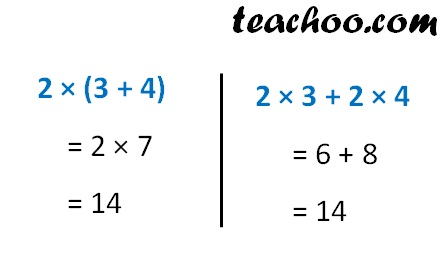Distributivity

Chapter 2 Class 6 Whole Numbers
Concept wise

If we add and then multiply or we multiply and then add,

This is called Distributivity

a × (b + c) = a × b + a × c

Example

For 2, 3 & 4Hence

2 × (3 + 4 ) = 2 × 3 + 2 × 4

Learn in your speed, with individual attention - Teachoo Maths 1-on-1 Class

### Transcript

Distributivity of Multiplication over Addition If we add and then multiply or we multiply and then add, We get the same answer This is called Distributivity a × (b + c) = a × b + a × c Example For 2, 3 & 4 Hence 2 × (3 + 4) = 2 × 3 + 2 × 4